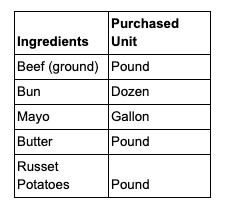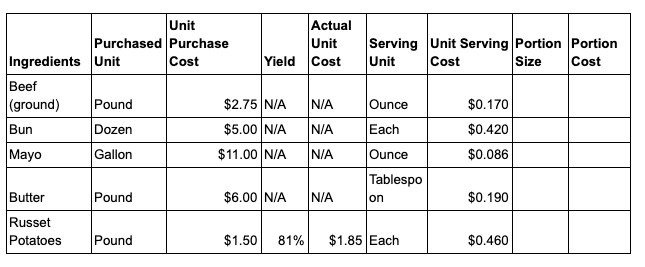### Calculating plate cost is one of the most important practices that any restaurant manager or owner can undertake. Knowing what a dish costs helps you make decisions in key areas, such as menu pricing as well as COGS and budget targets.

Yet, the reality is that many operators overlook the ongoing exercise of plate costing, because it can be a tricky and time-consuming process. Not only do you need to list and cost all ingredients for a dish, but you need to factor in yields, conversions, and density.

And, you not only need to do this for every item on your menu but also your prep recipes. Many chefs and restaurateurs might perform this exercise once; however, once is not enough. Recipe costs change regularly as the prices of ingredients rise and fall with market rates. With food costs steadily on the rise and the ever-present vulnerability of waste, theft, and price volatility, keeping close tabs on the margins of your menu items is a critical restaurant food cost control tactic.

Read on to learn more about:

### What Is Plate Cost?

Plate cost, also known as recipe or portion cost, is the cost of a single menu item. Plate cost is one of two food costs you need to manage.

The other is period cost — or the food cost for a specific period — which you can calculate using the COGS ratio.

For both types of food cost, you’ll need to calculate the food cost percentage. You can do this by dividing the total costs by total sales to get a ratio and multiplying it by 100 to get a percentage.

### Why Is Plate Cost Important?

Calculating your plate cost is crucial for many reasons:

1. Plate cost is an important consideration when pricing your menu. To arrive at a suitable margin—let’s say you want 20%—depends on knowing your costs. Remember, margin is simply the difference between the cost and the price. It is usually expressed as a percentage and calculated using this formula: Profit/Sales*100
2. Tracking how recipe costs change gives you timely information about when to raise your prices or change your menu or recipes. This is especially important as the price of ingredients regularly change based on seasonality and inflation.
3. It assists in engineering your menu for profits. Menu engineering consists of various actions you take to create a profitable menu, including pricing, design, placement, dish choice. For example, with menu engineering, you can find the right balance of low and high-margin dishes. You can even place high-margin dishes in the prime real estate area of the menu known as the Golden Triangle. This is the area of the menu where the diner’s eye naturally gravitates to first (middle, top right corner, and top left).
4. It helps you achieve cost targets. In addition to sales targets, you likely have cost targets. You’re more likely to reach these targets if you track changes to portion costs over time, as this tracking gives you ample time to take any corrective action. For example, if food costs increase for three months in a row, you can implement food cost controls or raise prices.

### How to Calculate Plate Cost

Now that you know what plate cost is and why it’s important, let’s look at how to calculate it using the following 3-step process:

Step 1: Create Standardized Recipes
Step 2: Build Your Recipe Costing Template
Step 3: Complete Your Recipe Costing by:

• Listing all ingredients
• Listing the purchased unit
• Listing the purchase cost
• Including the Yield %
• Calculating the actual unit cost
• Adding the serving unit of the recipe
• Calculating the unit serving cost
• Determining portion size
• Calculating portion cost

The inspiration behind the above process comes from Roger Fields’s book, Restaurant Success by the Numbers. In the book, he explains how to cost menu ingredients for a simple and complex dish.

### Step 1: Create Standardized Recipes

Don’t skip this step as recipes are your foundation for accurate recipe costing. Ensure you have recipe cards for all your dishes and store them in an accessible place for those who need it when preparing a meal.

Your recipe card will include:

• A list of ingredients
• Cooking time
• Preparation steps
• Units of measurement
• Weights

### Step 2: Create a Recipe Costing Template

Next, open a spreadsheet and create a table with the following 9 key headings:1.  Ingredients: A full list of ingredients used to make the dish.
2. Purchased unit: This is the unit of measure that the ingredients were purchased in. This can be anything from pounds and ounces to gallons and cases.
3. Unit purchase cost: The price per unit of measure as displayed on the supplier invoice.
4. Yield: Yield is expressed as a percentage and is the amount left after trimming and cleaning. For example, the usable weight after you’ve trimmed and cleaned a four-pound (64-ounce) steak may be three-pound (48 ounces).
5. Actual unit cost: The cost after you’ve factored in your yield.
6. Serving unit: The unit of measure used in the recipe.
7. Unit serving cost: The cost per serving unit, calculated using this formula: Cost per measure/units per measure
8. Portion or serving size: How much of each ingredient goes into a dish you serve customers. For example, a tablespoon of butter or 5 ounces of beef.
9. Portion cost: The cost of the serving size for that ingredient, calculated using the following formula: Portion size x unit serving cost.

### Step 3: Complete the Recipe Costing Spreadsheet

With your table complete, it’s time to cost a dish. To illustrate how to do this, let’s look at how to calculate the recipe cost for something simple: burger and chips.

First, list all the ingredients for the dish. For example:Second, list the purchased unit as per the supplier invoice. For example:Third, list the purchase cost of those ingredients as per the invoice. For example:Fourth, include your yield %. Your yield % will differ depending on the type of ingredient, but a good starting point is to use this Produce Yield Chart from Chef’s Resources, which details the average yield for certain whole products. For example, the yield for the Russet potatoes is 81%.Fifth, calculate the actual unit cost for each ingredient by applying the yield %. You only need to do this calculation for those ingredients that have a yield %.

In our example, this means calculating the cost of Russet potatoes. To make this calculation, take the cost per pound and divide it by the yield %. This brings the actual cost to \$1.85 (\$1.50/81%).Sixth, update your table with the serving unit used in your recipe. For example:Seventh, calculate your unit serving cost. This can be tricky as it involves several unit conversions. For example, if you purchase an item by the pound but use it by the cup, you’ll have to weigh a cup to arrive at the correct unit serving cost.

Let’s look at how to calculate the unit serving cost for each ingredient, using a simple formula: Cost per measure/units per measure. Keep in mind that for items with no yield percentage, the cost per measure will be the unit purchase cost. For items with a yield percentage, it will be the actual unit cost.

• Beef: Because the serving unit is an ounce and there are 16 ounces in a pound, the serving unit cost is \$0.17 (\$2.75/16).
• Bun: Twelve buns are \$5, so each bun is \$0.42 (\$5/12).
• Mayo: There are 128 ounces in a gallon, so one ounce of mayo costs \$0.086 (\$11/128).
• Butter: A tablespoon of butter weighs roughly 14.18 g or 0.5 ounces (half an ounce). This means there are 32 half ounces (or tablespoons) of butter in a pound. So, one tablespoon of butter costs \$0.19 (\$6/32).
• Russet potatoes: Let’s assume that your average potato is 4 ounces. This means that there are 4 potatoes in a pound, and your unit serving cost is \$0.46 (\$1.85/4). Remember, potatoes have a yield, so you’re dividing the actual unit cost by the units per measure.An often-overlooked measure during these calculations is density.

You’ll also need to factor in density because, for example, a pound of sugar will not be the same volume as a pound of flour.

Luckily, you don’t have to worry about this calculation if you use a density calculator. xtraCHEF provides a density calculator as part of its food cost management software.

Just let xtraCHEF know how much the ingredient weighs:The software will then store the density as a background variable to ensure more accurate costing.

Eighth, determine the portion size or how much of each ingredient will go into the dish. Refer back to your recipe here.Ninth, calculate your portion cost using this formula: Portion size x unit serving cost.Finally, tally the portion cost of all your ingredients and include a waste percentage. There will always be some waste that occurs during cooking, which you should factor in. Unfortunately, there’s no fixed waste factor percentage you can apply.

As Roger Fields explains: “The amount of waste will depend on the concept, preparation methods, and the skill level of your staff. In my restaurants, I would use between 10 and 15 percent depending on the menu item. Use a percentage that will allow you to price your menu competitively and still maximize profits.”

For this example, let’s assume a waste factor of 10%. This brings the total plate cost to \$2.207.You can now calculate your food cost percentage for that plate. Simply divide the selling price into the plate cost for the food cost ratio and multiply the ratio by 100 for the food cost percentage. For example, a selling price of \$6.50 gives you a food cost percentage of roughly 34% (\$2.207/\$6.50)*100.

### Your Plate Cost and Beyond

Calculating plate costs may be one of the most important practices for restaurant success as it helps with menu engineering and even pricing. Yet, it’s also one of the most arduous undertakings that you need to complete.

But it doesn’t have to be as long as you follow the right process: Create standard recipes, build your plate costing template, and complete your recipe costing by doing everything from listing ingredients and including yield to determining portion size and calculating the final portion cost.

From there, you can focus on other key areas like pricing your menu, having the right combination of dishes, and ensuring the correct placement to boost profits.

And remember: As simple as the above process is, calculating and keeping track of plate cost can still be a time-consuming and challenging process.

But if you invest in the right recipe management software, the process will feel that much easier because the software will help you:

• Crunch the numbers
• Track and compare costs
• Visualize profits thanks to dynamic interactive reports

xtraCHEF provides recipe management software that does precisely this.

Nick Darlington is a B2B writer who conceives, writes and produces engaging blog posts and lead magnets for technology companies. When he’s not writing for clients, he’s blogging at WriteWorldwide. See how he can help your business by visiting his site and scheduling a no-string attached call.